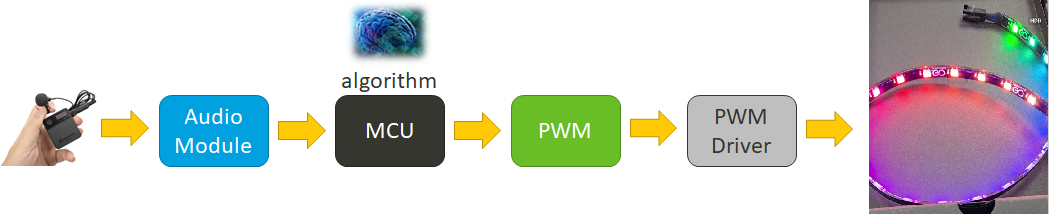# MediaTek Genio 130A Wi-Fi 6 多媒體互動情境燈光聖誕樹解決方案

MediaTek Genio 130A 整合 ARM® Cortex-M33 MCU 應用處理器與獨立音訊數位訊號處理器 ( DSP )  及 AI 引擎。使用本機語音命令或雲端語音處理套用氣氛模式設定排程與時間、播放音樂及燈光同步。

1. PWM initial

``static void PWM_init(void) {    int32_t total_count;    hal_gpio_init(HAL_GPIO_35);    hal_pinmux_set_function(HAL_GPIO_35, 3);    hal_pwm_init(HAL_PWM_6,HAL_PWM_CLOCK_26MHZ);    hal_pwm_set_frequency(HAL_PWM_6, 2000, &total_count);    hal_pwm_set_duty_cycle(HAL_PWM_6, 999);    hal_pwm_start(HAL_PWM_6);}``

2. Sound initialization
``ATTR_ZIDATA_IN_RAM static short record_buffer;constintbitdepth= 16;structmsd_hw_paramsparams;static sound_t*w_snd= NULL;static intvSound_init(void) {    {intret = 0;if (bitdepth!= 16 && bitdepth!= 32) {aud_error("bitdeptherror: %d", bitdepth);return 0;}params.format= bitdepth== 16 ? MSD_PCM_FMT_S16_LE : MSD_PCM_FMT_S32_LE;params.channels= 1;params.period_count= 4;params.period_size= 960;params.rate= 16000;intbytes_per_frame= bitdepth* params.channels/ 8;intdata_frames= params.rate;intdata_size= bytes_per_frame* data_frames;if (w_snd== NULL) {connect_route("UL3", "INTADC in", 1, CONNECT_FE_BE);connect_route("I_60", "O_14", 1, CONNECT_IO_PORT);connect_route("I_61", "O_15", 1, CONNECT_IO_PORT);ret = snd_pcm_open(&w_snd, "UL3", 0, 0);``

3. 取得麥克風接收音量峰值

``intget_max_volume(){inti=0;intmax = 0;intbytes_per_frame= bitdepth* params.channels/ 8;intdata_frames= params.rate;intdata_size= bytes_per_frame* data_frames;ret = snd_pcm_read(w_snd, record_buffer, data_frames);if (ret != data_frames) {aud_msg("ret: %d", ret);}for (i=0; i<data_frames; i++) {if (record_buffer[i] > max) max = record_buffer[i];}return max;}``

4. 計算環境聲音調整 PWM duty

``If (max volume > THRESHOLD) {PWM duty cycle = (PWM duty cycle < 1000 )? PWM duty cycle + 200 : 1000;} else If (max volume < THRESHOLD) {PWM duty cycle = (PWM duty cycle > 0)? PWM duty cycle 200: 0;}hal_pwm_set_duty_cycle(HAL_PWM_6, PWM duty cycle);``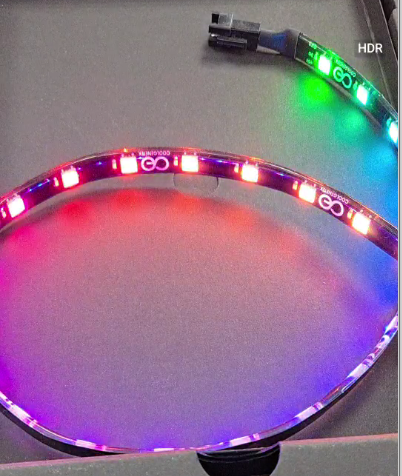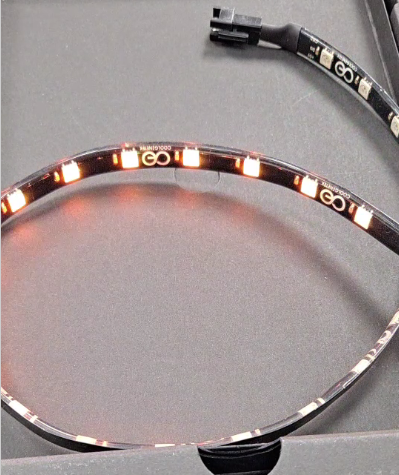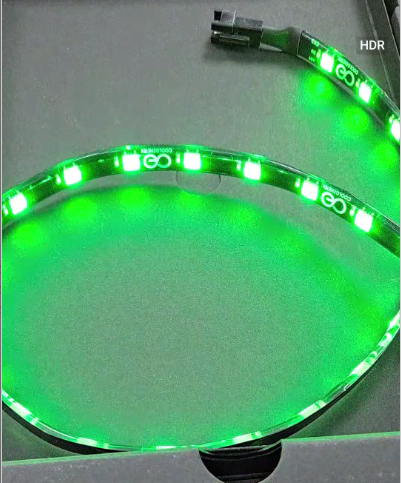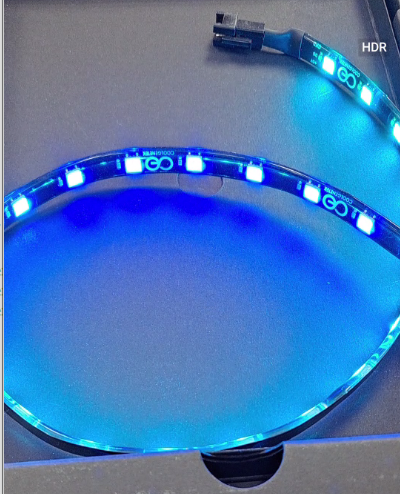#### ►場景應用圖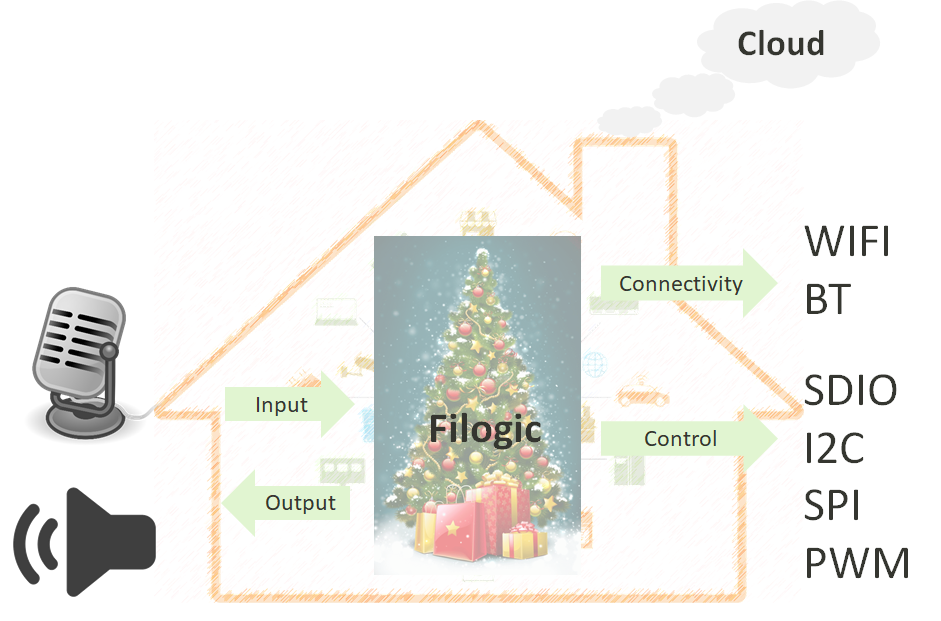#### ►展示板照片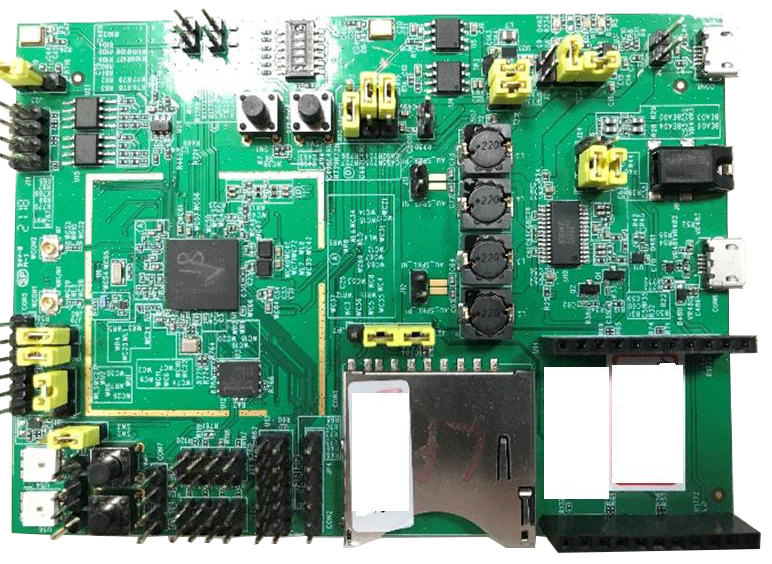#### ►方案方塊圖